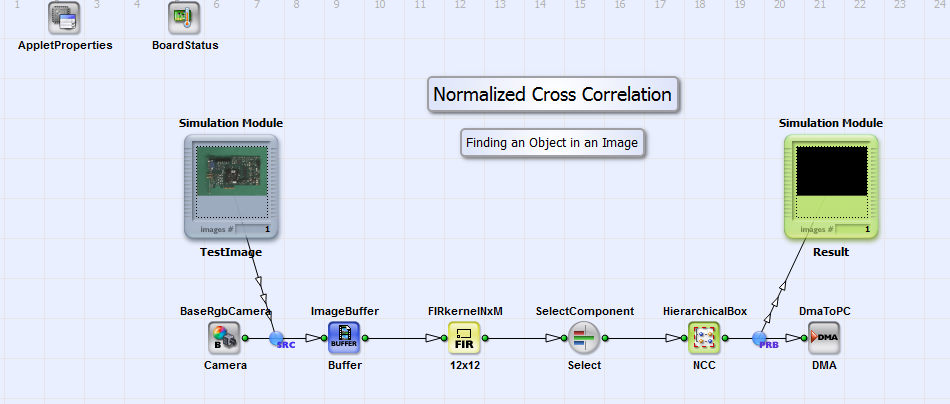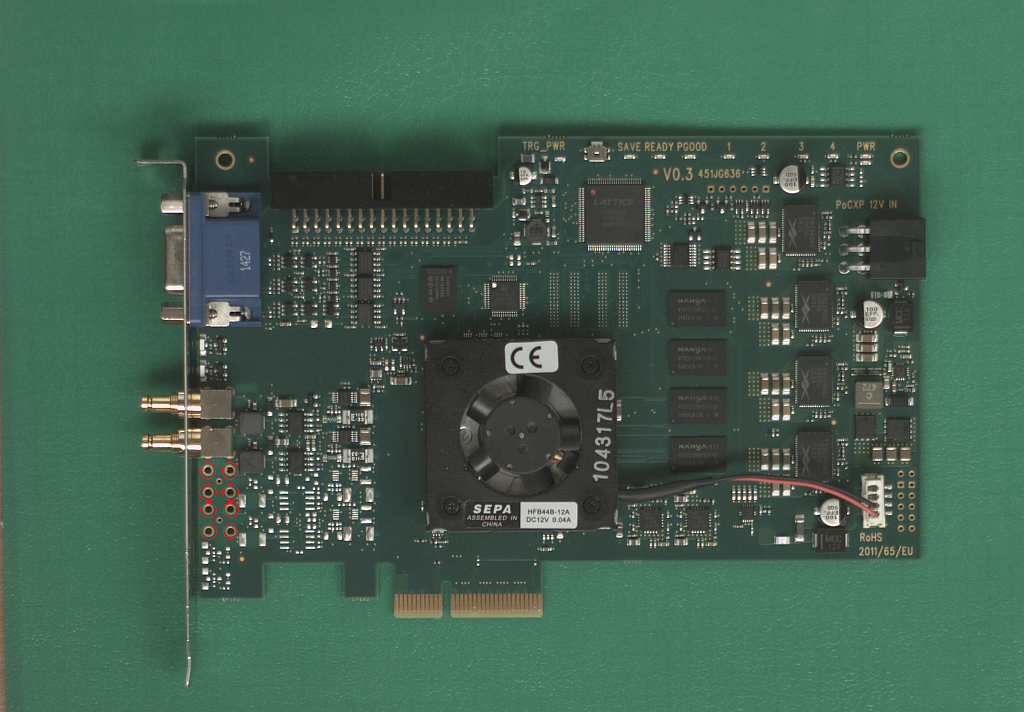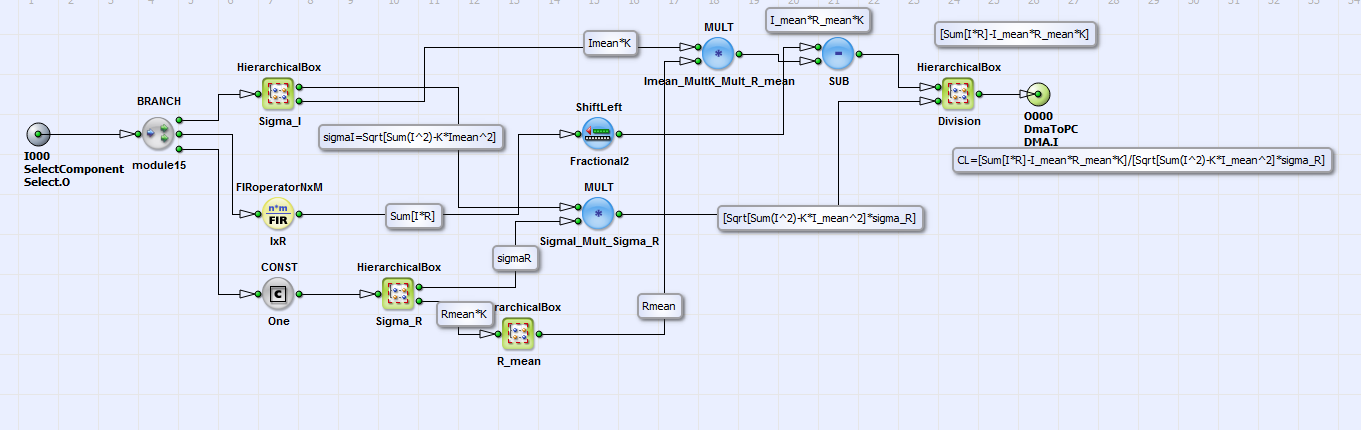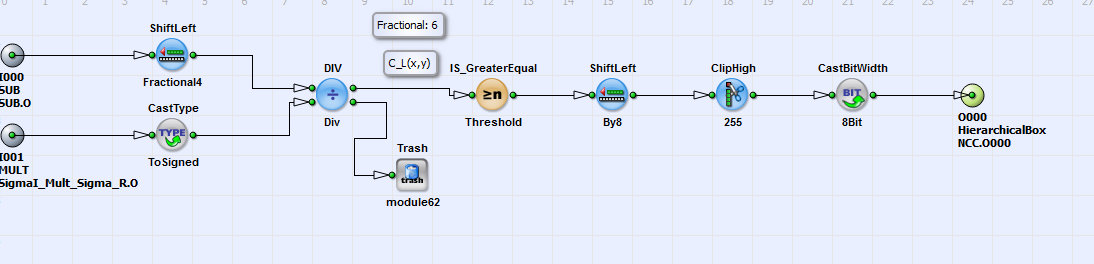#### Implementation in VisualApplets

In "NormalizedcrossCorreslation.va" an object is identified in an image using the NCC algorithm according to eq. 55. In Fig. 370 you can see the basic design structure of "NormalizedCrossCorrelation.va".Figure 370. Basic design structure of "NormalizedCrossCorrelation.va"

In an image from an RGB camera in Camera Link base configuration an image kernel of 12x12 pixels is defined with FIRkernelNxM operator 12x12. The kernel size depends on the object to be identified. The object is identical to the reference image in eq. 55. One color component (here: red) is selected with operator SelectComponent for identifying an object with the NCC algorithm according to eq. 55 in the HierarchicalBox NCC. As result a binary image with image size of original input image with value "1" at the identified object position and "0" at any other position is sent via DMA to PC. The example is configured for the RGB testimage "PCB.tif" which you find in the VisualApplets installation directory under examples\Processing\ObjectFeatures\NormalizedCrossCorrelation. This image has dimensions of 1024x712 pixels. In Fig. 371 the test image is shown.Figure 371. Test image "PCB.tif"

The six small holes on the lower left side of the PCB board are the objects to be identified in this example. The object dimensions are here 12x12 pixels. In the design in box NCC (see Fig. 370) the algorithm according to eq. 55 for locating these objects is implemented. Please see the comment boxes beside the links and HierarchicalbBoxes for further information on the corresponding equation part. In Fig. 372 you can see the content of HierarchicalBox NCC.Figure 372. Content of HierarchicalBox NCC

You can adapt the design example to your image and objects of choice as explained in the following. If you want to use grayscale images instead of RGB images just replace operator BaseRgbCamera with operator BaseGrayCamera and delete operator SelectComponent.

1. Adapt the size of operator FIRkernelNXM_12x12 to the dimensions of the objects you want to identify in the image.

2. With "right-mouse-click" on HierarchicalBox NCC under "Properties" you can set the number "K" of pixels of the object to be found. With object size of 12x12, K is 144. Under "Properties" you can also adapt the binarization threshold. This threshold is used to visualize the objects position with "1" in the result image.

3. Adapt the number of kernel columns and rows to the object dimension at the output of CONST operator One in HierarchicalBox NCC.

4. Load pixel value file of the object to be identified (which is ) to FIRoperatorNxM IxR in HierarchicalBox NCC. You can use program "NCC.m" to create this file ("R.txt").

5. Load the same file to the FIRoperatornxM Rmean_Mult_K in HierarchicalBox Sigma_R in HierarchicalBox NCC.

6. Load the file "Rpower2.txt" (created with Matlab program "NCC.m") to the FIRoperatorNxM Sum_R_R in HierarchicalBox Sigma_R. The content of this file are the pixel values of the object (divided by 2) to the power of two .

In the HierarchicalBox Division (see Fig. 372) the final result of eq. 55 is calculated with the division operation DIV. You can see the content of box Division in Fig. 373.Figure 373. Content of HierarchicalBox Division

The division is performed with six fractional bits i.e. the result of is between -64 and 64 instead of - 1 and 1. To visualize the position of the identified object the result image is binarized with IS_GreaterEqual operator Threshold. The threshold value is set to 50 in this example. You can vary this value directly in the operator or as explained above with "right-mouse-click" on box NCC. The result image which is sent to PC is shown in Fig. 374. The pixels at position of the small holes on the lower left side of the PCB board (see Fig. 371) obtain value "1" and all other pixels "0". The result image is sent via DMA to PC. Instead of this it can also be input for further image processing steps.Figure 374. result image with "1" at object positions (zoomed view)